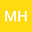•The aim of this work is to study qualitative dynamical properties of a generalized hepatitis B epidemic model and its dynamically consistent discrete model. Positivity, boundedness, the basic reproduction number and asymptotic stability properties of the model are analyzed rigorously. By the Lyapunov stability theory and the Poincare-Bendixson theorem in combination with the Bendixson-Dulac criterion, we show that a disease-free equilibrium point is globally asymptotically stable if the basic reproduction number $\mathcal{R}_0 \leq 1$ and a disease-endemic equilibrium point is globally asymptotically stable whenever $\mathcal{R}_0 > 1$. Next, we apply the Mickens’ methodology to propose a dynamically consistent nonstandard finite difference (NSFD) scheme for the continuous model. By rigorously mathematical analyses, it is proved that the constructed NSFD scheme preserves essential mathematical features of the continuous model for all finite step sizes. Finally, numerical experiments are conducted to illustrate the theoretical findings and to demonstrate advantages of the NSFD scheme over standard ones. The obtained results in this work not only improve but also generalize some existing recognized works.